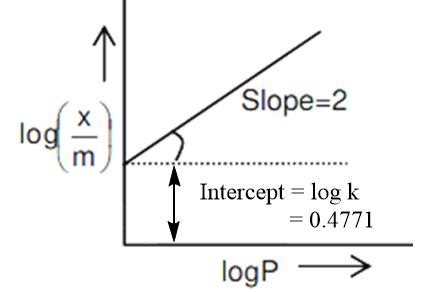Surface phenomenon
Question

# For Freundlich adsorption isotherm, a plot of log (x/m) (y-axis) and log P (x-axis) gives a straight line. The intercept and slope for the line is 0.4771 and 2, respectively. The mass of gas, adsorbed per gram of adsorbent if the initial pressure is 0.04 atm is _________ × 10-4 g.(log 3 = 0.4771)

Moderate
Solution

##$\left(\frac{x}{m}\right)=k\left(P{\right)}^{\frac{1}{n}}$Intercept $\mathrm{log}k=0.4771$$=48×{10}^{-4}\mathrm{g}$

Get Instant Solutions